## ML Aggarwal Class 8 Solutions for ICSE Maths Chapter 5 Playing with Numbers Ex 5.2

Find the values of the letters in each of the following and give reasons for the steps involved (1 to 11):
Question 1.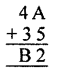Solution: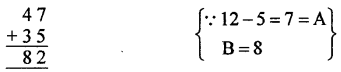A = 7, B = 8

Question 2.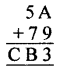Solution: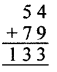9 + 4 = 13, ∴ A = 4
B = 1 + 5 + 7 = 3
C = 1
A = 4, B = 3, C = 1

Question 3.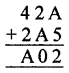Solution:5 + 7 = 12, ∴ A = 7
1 + 2 + 7 = 10
1 + 4 + 2 = 7 = A

Question 4.Solution:A = 4 or 9
A ≠ 4 as A + A = A, 4 + 4 ≠ 4
A = 9
B = 1
∴ Hence A = 9, B = 1

Question 5.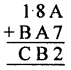Solution:5 + 7 = 12
∴ A = 5
B = 4, C = 6
Hence, A = 5, B = 4, C = 6

Question 6.Solution:B = 3 or 8
If B = 8
1 + 1 + 7 = 9 then A = 7
C = 4 – 2 = 2
7 + 1 = 8 = B
Hence, A = 7, B = 8, C = 2

Question 7.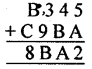Solution: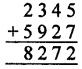A = 7 (∵ 5 + 7 =12)
1 + 4 + 2 = 7(A)
∴ B = 2
1 + 2 + 5 = 8, C = 5
Hence, A = 7, B = 2, C = 5

Question 8.Solution: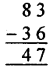∴ B – 6 = 7
∴ B = 3
A – 1 – B = 4
A – 1 – 3 = 4, A = 4 + 4 = 8
Hence, A = 8, B = 3

Question 9.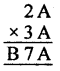Solution: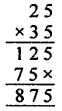A = 1 or 6 or 5 as 1 × 1 = 1
or 6 × 6 = 6
or 5 × 5 = 5
Taking A = 5
∴ B = 8
Hence, A = 5, B = 8

Question 10.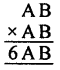Solution:B × B = B
B = 1, 6, 5
If B = 5, and A = 2, then
25 × 25 = 625
Hence, A = 2, B = 5

Question 11.Solution: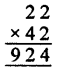A = 2 or 8
Let A = 2
Hence, A = 2

Question 12.
Fill in the numbers from 1 to 6 (without repetition) so that each side of the magic triangle adds up to 12.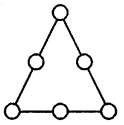Solution:
Numbers 1 to 6 without repetition
The sum from each side = 12
There can be much more solutions such as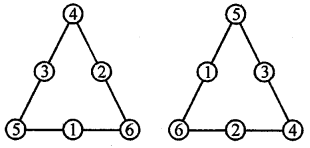Question 13.
Complete the magic square given alongside using number 0, 1, 2, 3, ……., 15 (only once), so that sum along each row, column and diagonal is 30.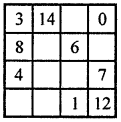Solution:
In the magic square given use of number 0, 1, 2, 3, ……….., 15
only once so that the sum along each row, column and diagonal is 30.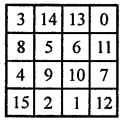3 + 8 + 4 = 15 + 15 = 30
3 + 5 + 10 + 12 = 30
3 + 14 + 0 = 17 + 13 = 30
0 + 6 + 9 + 15 = 30
0 + 7 + 12 = 19 + 11 =30
15 + 1 + 12 = 28 + 2 = 30
8 + 6 + 11 = 25 + 5 = 30
13 + 6 + 1 = 20 + 10 = 30
14 + 5 + 2 = 21 + 9 = 30

Question 14.
Fill in the blanks to complete the following number triangle:Solution: